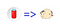# ES6 Cool stuffs — A big fat ArrowYup, if input is a can of Cola, return value is a fat unicorn. Sounds logical, doesn’t it? 😆

# Function in JS

`function HelloWorld(){ console.log('Hello World');}HelloWorld(); //'Hello World'`
`let sayHello = function() { console.log('Hi there, do you come here often? ')`
`let sayHello = new Function(`console.log('Hello World');`);sayHello(); //'Hello World';`

# Arrow function expression

## Basic

`//Several parameters(param1, param2, ..., paramN) => { //statements }//No parameter() => { //statements }`
`let HelloWorld = () => { console.log(‘Hello World’) }`
`let sumUp = (a, b) => a + b //OKlet sumUp = (a, b)=> a + b                    //NOT OKlet sumUp = (a, b) =>a + b                       //OK`
`let HelloWorld = () => console.log('Hello World')`
`let sayMyName = () => `Maya Shavin`sayMyName() //"Maya Shavin"`
`let getObject = () => { greet: function(){ console.log('hi') }}getObject() //SyntaxError: Unexpected token '('let getObject2 = () =>({ greet: function(){ console.log('hi') }})getObject2().greet() //hi`
`let printName = name => console.log(name)`

## `Binding 'this'`

`let WaitAndSee = {    counter: 1,    wait: function(){        let numbers = [1,2,3,4]        numbers.map(function(x) {             this.counter++        })    },    see: function(){        console.log(`Counter: \${this.counter}`)        console.log(`Window counter: \${window.counter}`}}`
`window.counter = 1WaitAndSee.wait()WaitAndSee.see()`
`let WaitAndSee = {    counter: 1,    wait: function(){        let numbers = [1,2,3,4]        numbers.map(x => this.counter++)    },    see: function(){        console.log(this.counter)    }}WaitAndSee.wait()WaitAndSee.see() //5`

## No ‘arguments’ object

`let showArguments = () => console.log(arguments)showArguments() //ReferenceError: arguments is not defined`
`let showArguments = (...arguments) => console.log(arguments)showArguments() //[]`

## Using as constructor?

`function WaitAndSee(){...} //OKlet WaitAndSee = () => {} //OKWaitAndSee() => {} //Wrong syntax`
`let WaitAndSee = function(){}console.log(WaitAndSee.prototype) //{constructor: f}let WaitAndSee2 = () => {}console.log(WaitAndSee2.prototype) //undefined`

## Non-method function

`let WaitAndSee = {    counter: 1,    wait: () => { this.counter++ },    see(){        console.log(this.counter)        console.log(window.counter)    }}window.counter = 1WaitAndSee.wait()WaitAndSee.see() // 1, 2 - window.counter is updated instead!`

# Pros

`let request = fetch("")              .then(function(response){...})              .then(function(result){...})              .then(function(data){...})              .catch(function(error){...})`
`let request = fetch("")             .then(response => {...})             .then(result => {...})             .then(data => {...})             .catch(error => {...})`

# Cons

`let getMyName = () => `Maya Shavin``

Written by